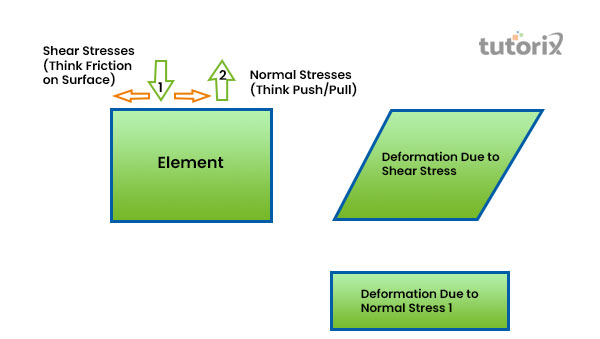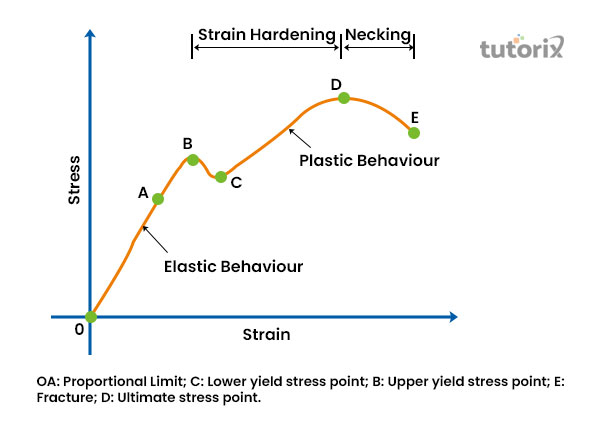# Solid Deformation

## Introduction

The object or solid shape is the matter of geometrical description of the space that has been acquired by that object and is denoted by its external boundaries. Due to the application of physical forces, the shape of the solid changes and this phenomenon is denoted as the solid deformation.

Objects that are under certain external forces undergo deformation by twisting, pulling apart, and cracking, compressing, and squashing. In Physics, the process of deformation of objects, or solids can be described by two terms: Strain and Stress.

The relation between these Strain and Stress can be written in the form:

Stress = Elastic Modulus × Strain

## What is deformation?

• The physical stress due to which an object changes its size and shape is called deformation. The physical forces that bend, stretch, crack, compress, and pull apart the objects are gravity and weight.
• The activity and effects of the physical forces upon objects totally depended on their physical composition (Cui et al. 2017).
• Thus, when a brick wall is found with a crack then it can be stated that a certain amount of strains and stresses have been acted upon the wall. Two common types of deformation are faults and folds. There are also several types of rocks or solid deformation like ductile deformation, elastic deformation, and brittle deformation.

## Deformation of solid: Explanation

If the shape, length, or volume of a solid material gets changed due to the application of external forces, mechanical forces, or even physio-chemical forces, then this phenomenon is considered to be the deformation of solid (Xu et al. 2017). The solid materials’ shape is determined by the geometrical description of the scope that the solid has occupied and is considered its external boundaries.Figure 1: Deformation of metals

Three main types of deformation take place in solid materials like elastic, brittle, and ductile deformation.

• Elastic deformation is the temporary deformation that disappears when the external forces are removed from the solid materials (Zhao et al. 2020).
• Plastic or brittle deformation is the permanent deformation that is irreversible and remains even after the force is removed from the solid.
• The solid objects’ shape or volume is changed permanently due to ductile deformation.

### Elastic and plastic deformation of solid

There are several types of deformations, among which the elastic deformation and plastic deformation are the major ones. Plastic deformation is also determined as brittle or permanent deformation (Gcekjr, 2020). This plastic deformation is basically irreversible and is one of the types of deformation that remains even after the applied forces have been removed from the objects or solid materials. Elastic deformation is considered the temporary deformation and is also irreversible. This kind of solid deformation vanishes after the exerted forces are removed from the objects or solids (Xu et al. 2017). If an object is subjected to external forces that material will experience elastic deformation that is followed by the plastic or brittle deformation.

## Stress and Strain

Stress is the physical quantity that defines the external pressures magnitude, which is the main cause of deformation. On the other side, Strain deals with the measurement of deformation degrees. The relation between Strain and Stress can be stated as,

Stress = Elastic Modulus × Strain (Ren et al. 2017).

Elastic modulus is determined as the ratio between this strain and stress and describes a certain amount of pressure exerted upon per deformation unit.Figure 2: Strain and Stress: Curve

There are several types of stresses such as shear stress, bulk stress, tensile stress, and compressive stress. Strain deals with an explanation of the fractional change taking place in the solid materials like in their geometry, volume, or length and is considered as the dimensionless number.

## Conclusion

Deformation of solid is denoted as the change of shape, volume, length, and other properties of the solid materials after the application of mechanical, physical, or physio-chemical forces. The solid deformation process is defined by two terms in physics those are stress and strain (Xu et al. 2017).

Several types of solid deformation include, Elastic or temporary deformation; plastic or brittle deformation is determined as the permanent deformation; and another is ductile deformation, where the shape or volume of solid materials get change permanently. The elastic and plastic deformations are irreversible in nature.

## FAQs

Q1. What do you mean by deformation of metals?

If the metal body changes its shape, size, or volume, after a certain physical force is exerted on it then it is determined as the metal deformation. This deformation can be caused by external forces, by mechanical actions, or even by physio-chemical processes.

Q2. What is length deformation?

The modulus of the length deformation is called the Young’s Modulus. It is denoted as the slope of the line arranged for an elastic region of the strain and stress graph. It generally deals with the measurement of how much the material has been stretched.

Q3. What are restoring and deforming forces?

If an external force is put on a solid material it will produce a change in its actual shape, position or size. This phenomenon is denoted as a change in length, shape or volume, which takes place in a body after the application of deforming force. The force that gradually restores the shape of the solid material by removing the deforming force is determined as the reforming force.

Q4. What is the use of Stress and Strain curve?

Stress is a deforming force that an object experiences per unit area. Strain is the change in quantity per unit of that object's actual value. The Stress and Strain curve, deals with the materials’ behaviour when an external pressure is mostly applied to it and changes in its volume, shape, or length occur.

## References

### Journals

Cui, Y., Chan, D., & Nouri, A. (2017). Coupling of solid deformation and pore pressure for undrained deformation—a discrete element method approach. International Journal for Numerical and Analytical Methods in Geomechanics, 41(18), 1943-1961. Retrieved from: www.researchgate.net

Ren, Y. M., Lin, X., Fu, X., Tan, H., Chen, J., & Huang, W. D. (2017). Microstructure and deformation behaviour of Ti-6Al-4V alloy by high-power laser solid forming. Acta Materialia, 132, 82-95. Retrieved from: www.researchgate.net

Xu, Q., Jensen, K. E., Boltyanskiy, R., Sarfati, R., Style, R. W., & Dufresne, E. R. (2017). Direct measurement of strain-dependent solid surface stress. Nature communications, 8(1), 1-6. Retrieved from: www.nature.com

Zhao, Y., & Borja, R. I. (2020). A continuum framework for coupled solid deformation–fluid flow through anisotropic elastoplastic porous media. Computer Methods in Applied Mechanics and Engineering, 369, 113225. Retrieved from: www.sciencedirect.com

Website